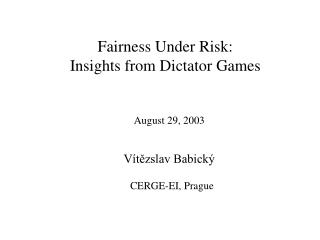DownloadDownload PresentationCERGE-EI, Prague

# CERGE-EI, Prague

Télécharger la présentation## CERGE-EI, Prague

- - - - - - - - - - - - - - - - - - - - - - - - - - - E N D - - - - - - - - - - - - - - - - - - - - - - - - - - -
##### Presentation Transcript

1. Fairness Under Risk: Insights from Dictator Games CERGE-EI, Prague August 29, 2003 Vítězslav Babický

2. MOTIVATION Existing theories and experiments – decisions about ex-ante known pies, possibly with information constraint. People’s life circumstances are diverse and subject to chance events (even after decisions made – shares are distributed, prenuptial agreement is signed) So, let’s introduce risk into decision making process (and let’s stick to use of the expected utility theory first)

3. VARIATION ON DICTATOR GAME The dictator game is the simplest one – the decision is affected only by preferences of the decision-maker – we can study a single agent’s preferences separately RISK: Only a probability distribution of the pie size is known to the dictator at the moment of decision. The decision is to give a percentage share of the actual pie to the recipient.

4. Theory of fairness, competition and cooperation [FCC] (Fehr, Schmidt, 1999) Proposed model: aversion to differences in payoffs Utility function in the form Ui(x) = xi – (i/(n–1))∑kmax(xi–xk,0) – – (i/(n–1))∑kmax(xk–xi,0) n=number of players (=2 for the dictator game) Empirical results for various classes of games can be explained in accordance with this fairness model

5. Theory of Equity, Reciprocity and Competition [ERC] (Bolton, Ockenfels, 2000) Agents maximize Vi = Vi(yi, i) where yi is their payoff and i = i(yi, c, n) is the relative payoff (c = yi total payoff) i = yi/c if c > 0 and 1/n otherwise Under this utility function agents have narrow self-interest: assumptions Vi1 0, Vi11 0, Vi22< 0, Vi2= 0 for i = 1/n

6. Examples: Vi(ci, i) = aici – bi/2(i – 0.5)2 ai, bi >0 given constants Additively separable Vi(yi, i) = u(yi) – v(i) u concave, v convex – given functions Works for Dictator game (and many others) Also works under incomplete information: Response to proposal in the ultimatum game is done without knowing actual size of the pie

7. THEORY MODIFICATIONS Need: Utility concave For the FS model, I can introduce exponent γ>1 and a concave function u into (note: higher share is always preferred to lower share by dictator, i.e. xdictator≥xrecipient always holds) Ui(x) = u(xi) – i (xi-xk)g Let C be random variable for the size of pie and p be the decision of the dictator – the share on the pie transferred to the recipient

8. The dictator solves maxp Eu((1–p)C) – E((1–2p)C)g F.O.C.: dEu((1–p)C))/dp+2γ(1–2p)g-1E(Cg)=0 S.O.C. valid whenever u is concave For simplicity, let u(x)=sgn(r) xr , r<1, r≠0 be an increasing concave CRRA function

9. The solution: (1–2p)g-1(1–p)1-r = r.sgn(r)E(Cr)/(2γE(Cg)) Left-hand side is always decreasing in p (γ>1, r<1), i.e. the dictator giving is higher if the right-hand side is lower (the decision depends on parameters γ, r) If EC1=EC2 then E(Ci2) determines variance of Ci (since Var Ci = E(Ci2) – [E(Ci)]2) Obviously, Var C1< Var C2 iff E(C12) < E(C22). Typically, also E(C1g) < E(C2g) for any γ>1; E(C1r) > E(C2r) for any r in (0,1) and E(C1r) < E(C2r) for all r<0 (namely for symmetric distributions)

10. For the ERC model, I can go through a similar analysis: Note: V(x, ) = sgn(r) xr – k (i–0.5)g = sgn(r) xr – k (2i–1)g/2g = sgn(r) xr – k’ (i–k)g Dictator maximizes EV(p)= sgn(r) (1–p)r E(Cr) – k(1–2p)g The solution: (1–2p)g-1(1–p)1-r = r.sgn(r)E(Cr)/(2kγ)

11. Now, the comparative statics is more obvious: (reminder: the left-hand side is decreasing in p) Dictator giving p is higher for lower variance treatments if r<0, it goes in the opposite direction if r in (0,1)

12. Remark: r=0 … the corresponding CRRA function is u(x)=log x Then, the dictator maximizes expected utility EV(p)= log(1–p) + E(log C) – k(1–2p)g i.e. the solution does not depend on changes in the size of pie to be distributed in this situation.

13. PILOT EXPERIMENT Dictator game with ex-ante unknown size of pie in two groups. Both have the same expected size but different variance (note: symmetric distributions were used). Subjects asked to decide both absolute and relative part of the pie to transfer to the second player. They are also asked about the preference of one of the decisions.

14. THEORETICAL PREDICTION: Dictator giving is (in relative terms) higher under low variance treatment for coefficients of relative risk aversion below -1 Dictator giving is (in relative terms) lower under low variance treatment for coefficients of relative risk aversion above -1 Dictator giving in relative terms is the same no matter the risk for risk-neutral agents and for agents with CRRA equal to -1

15. MORE INTUITION: Relative giving is preferred over absolute giving (and totally selfish agents are indifferent between both ways)

16. RESULTS (Prague) (25 subjects, CERGE 1st year students): For group A (low variance, 13 subjects): 4 decisions for absolute offer (risk captured by proposer) Average offers: 2108 absolutely 28% relatively (2506 expected value) For group B (high variance, 12 subjects): 1 decisions for absolute offer Average offers: 1425 absolutely 17% relatively (1533 expected value)

17. CONCLUSION Attitude to fairness is related with attitude to risk Theory suggests that changes in dictator giving under risky conditions correspond with opposite changes under increasing sure pie The observations (the pilot experiment) suggest that the coefficient of relative risk aversion is below -1, on average.